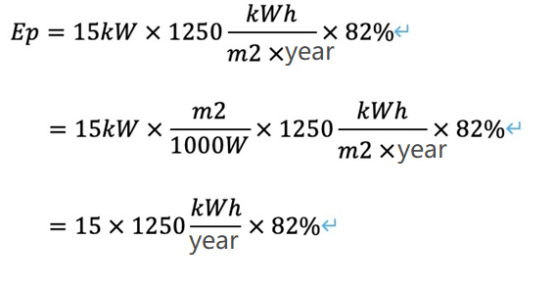# Photovoltaic power generation calculation

###### The power generation forecast of PV power station should be calculated and determined based on the solar energy resources at the site and taking into account various factors such as PV power station system design, PV array arrangement and environmental conditions.

The average annual power generation capacity Ep of PV power station is calculated as follows:

Ep=HA*PAZ*K

Ep: feed-in power generation (KW-h).

HA : total annual irradiation of horizontal solar energy (KW-h/m2).

PAZ : installed capacity of the system (KW).

K: is the integrated efficiency coefficient.

Photovoltaic power generation = installed capacity * light irradiation * integrated efficiency

• Installed capacity: the number of installed modules * peak power of each module.

(Attention: the power of different module sizes and types of modules may vary.)

• Light irradiance: the energy of sunlight, more sunlight is good, more light irradiance.
• Integrated efficiency:the efficiency of the received light into electricity, can be understood as the conversion efficiency. It is related to system design, equipment and so on. Also often referred to as "system efficiency".

For example:

15KW PV power system installed in Shenzhen, assuming that the tilted surface of the Shenzhen side of the light is 1250kWh/m2/year, the integrated efficiency of 82%, then the power generation of this system is：

Ep = 15 * 1250 * 82% = 15375KWh. Shenzhen installed a 15kW system can send 15375 degrees of electricity a year. Of course, the PV system will have decay, power generation will decline year by year.

Note:

Component 1W (watt) is measured in the irradiance 1000W/m2, cell temperature 25 degrees in the standard test environment, the above formula can be understood as follows:PV people often say:

equivalent hours = light * system efficiency

if the equivalent hours is 1100, then 1kW PV system, the annual power generation is 1000 degrees of electricity, this is also the easiest  calculation method.